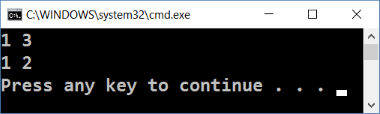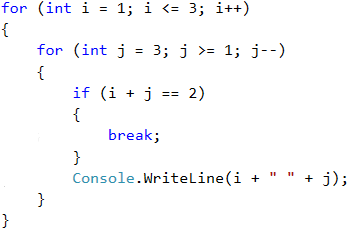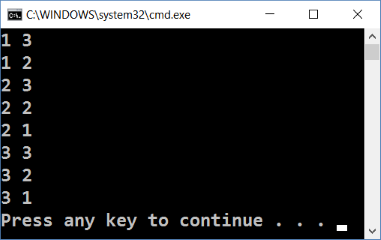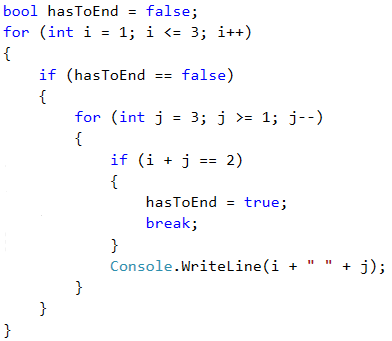# Nested Loops and Break

Once we have learned what the nested loops are and how the break operator works, it is time to figure out how they work together. For a better understanding, let's step by step write a program that should make all possible combinations of pairs of numbers. The first number of the combination is increasing from 1 to 3 and the second one is decreasing from 3 to 1. The problem must continue running until i + j is not equal to 2 (i = 1 and j = 1).

The desired result is:## Wrong Implementation

Here is a wrong solution that looks right at first sight:If we leave our program that way, our result will be as follows:Why is it so? As we can see, the result is missing "1 1". When the program reaches that point that i = 1 and j = 1, it enters the if check and executes the break operation. This way, it goes out of the inner loop, but then continues the execution of the outer loop. i grows, the program enters the internal loop and prints the result.When we use the break operator in a nested loop, it interrupts the execution of the inner loop only.

## Correct Implementation

What is the right solution? One way to solve this problem is by declaring a bool variable to keep track if the loop iteration has to continue. If you need to exit (leave all nested loops), we set the variable to true and exit the inner loop with a break, and in the next check we exit the outer loop. Here is an example implementation of this idea:Thus, when i + j = 2, the program will set the hasToEnd = true and exit the inner loop. Upon the next rotation of the outer loop, through the check, the program will not be able to reach the inner loop and will interrupt its execution.